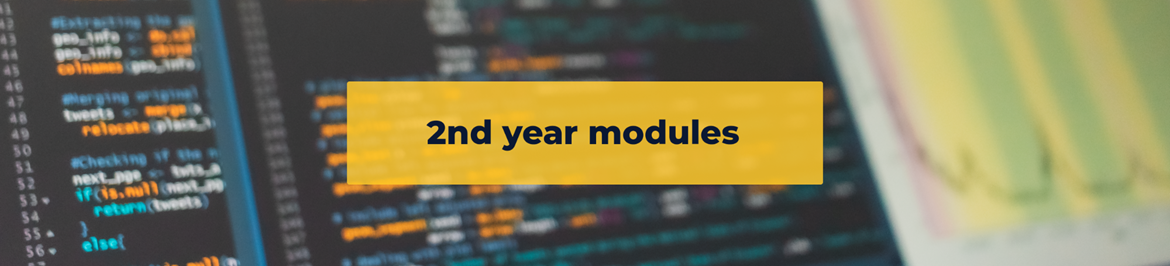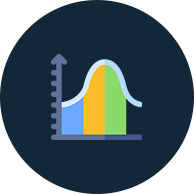# Mathematical Statistics 2

This course consists of the two modules STAS201 and STAS203 which must be passed separately.### STAS201

This module consists of 84 single lectures, 28 practicals and 28 tutorials during the first semester.

Pre-requisites

A previous pass in STAS101 and STAS102 and MATH104

Content

Basic probability concepts. Theory of continuous probability distributions. Expected values and MGF. Special continuous probability distributions: Uniform, Gamma, Exponential, Weibull, Pareto, and Normal. Theory of multivariate discrete and continuous distributions, Marginal and conditional distributions. Covariance and correlation. Theory of conditional expectation and conditional variance. Functions of Random variables: distribution function technique, transformation methods, MGF technique. Limiting distributions and stochastic convergence. CLT and applications. Sampling distributions: Chi-square, t, F, Beta distributions.### STAS202: Regression Analysis and Advanced Regression Topics (20 credits)

This module consists of 84 single lectures, 28 practicals and 28 tutorials during the second semester.

Pre-requisites

STAS/STATT101, STAS/STAT102, MATT102 OR STAV/STAE102, MATS101, MATS102 OR STAV/STAE102, MATT102

Content

1. Simple linear regression: The theoretical (mathematical) model, assumptions, estimation, coefficient of determination, prediction, regression through the origin.

2. Multiple linear regression: The model, assumptions, estimation, inferences. Polynomial regression, Second and higher order regression. Testing portions of a model. Stepwise regression. Models with qualitative and quantitative predictors. Model building. Regression pitfalls. Residual analysis. Piecewise regression. Inverse prediction. Weighted least squares regression. Qualitative response variables. Logistic regression. Log-Linear Models. Ridge regression. Robust regression. Model validation.### Statistics 2

Statistics 2 consists of the two modules STAS202 and STAS203 which must be passed separately.

STAS211: Probability, Distribution Theory and Estimation (20 Credits)

This module consists of 84 single lectures, 28 practicals and 28 tutorials during the first semester.

Pre-requisites

A previous pass in STAV101 - 102 and either MATA101 – 102 or MATH101 - 104.

Content

1. Probability concepts: Experiment, sample space and events.

2. Computing probabilities: Permutations and combinations. Conditional probability. Bayes' Theorem. Functions associated with random variables.

3. Special Discrete Distributions: Uniform, Bernoulli, Binomial, Hypergeometric, Poisson and Negative Binomial.

4. Special Continuous Distributions: Uniform, Exponential, Gamma, Chi-squared, Beta and Normal. Statistical Inference: Estimation and Hypothesis testing.